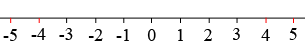SEARCH HOMEMath Central Quandaries & QueriesQuestion from Anshika, a student: Write rational number between -4 and -5Hi Anshika,

A rational number is a number that can be written $\large \frac{a}{b}$ where are $a$ and $b$ are integers and $b \ne 0.$Write a number that is between 4 and 5. Is it a rational? Write a rational number between -4 and -5.

PennyMath Central is supported by the University of Regina and the Imperial Oil Foundation.